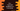# C++ program to find the smallest and the second smallest numbers## Find the smallest and the second smallest numbers in an array in C++:

We will learn how to find the smallest and second smallest numbers in an array of numbers in C++. For example, if the array is [1, 3, 5, 7, 2], the smallest number will be 1 and the second smallest number will be 2.

We can solve it in different ways. One traditional approach is to use two variables to hold the smallest and second smallest numbers and update the values with a loop. Another way is to sort the array and pick the first and the second element from the sorted array. Let’s learn how to write the programs for both of these approaches.

### Method 1: By using a loop:

The program will follow the below steps:

• Initialize two variables to hold the smallest and second smallest numbers.
• Assign INT_MAX to both of these variables. So, the smallest and the second smallest values will be the maximum integer value when the program starts.
• Start a loop from the first element to the end of the array. On each iteration, compare the current value pointed by the loop with the smallest and second smallest variables.
• If it is smaller than both of these variables, assign the smallest variable value to the second smallest variable and assign the current number to the smallest variable.
• If it is smaller than the second smallest variable, assign it to the second smallest variable.
• Once the loop ends, print the smallest and second smallest variables, which are the required values.
``````#include <iostream>
#include <climits>
using namespace std;

int main()
{
int arr = {1, 4, 2, 7, 4, 12, 0, 9};
int smallest = INT_MAX;
int secondSmallest = INT_MAX;

for (int i = 0; i < size(arr); i++)
{
if (arr[i] < smallest && arr[i] < secondSmallest)
{
secondSmallest = smallest;
smallest = arr[i];
}
else if (arr[i] < secondSmallest)
{
secondSmallest = arr[i];
}
}

cout << "Smallest value: " << smallest << endl;
cout << "Second smallest value: " << secondSmallest << endl;
}``````

The arr is the given array of integers. It is following the same steps we have discussed above. It will print:

### Method 2: By using loop and user input values:

We can take the numbers as inputs from the user. The following program takes the numbers as inputs and insert these numbers to the array before it finds the smallest and second smallest values:

``````#include <iostream>
#include <climits>
using namespace std;

int main()
{
int size, arr;
int smallest = INT_MAX;
int secondSmallest = INT_MAX;

cout << "Enter the size of the array: " << endl;
cin >> size;

for (int i = 0; i < size; i++)
{
cout << "Enter the number for index " << i << ": ";
cin >> arr[i];
}

for (int i = 0; i < size; i++)
{
if (arr[i] < smallest && arr[i] < secondSmallest)
{
secondSmallest = smallest;
smallest = arr[i];
}
else if (arr[i] < secondSmallest)
{
secondSmallest = arr[i];
}
}

cout << "Smallest value: " << smallest << endl;
cout << "Second smallest value: " << secondSmallest << endl;
}``````

If you run this program, it will give results as below:

### Method 3: By sorting the array elements:

If we sort the array elements in ascending order, the first element of the sorted array will be the smallest element and the second element will be the second smallest element of the array. We can use the inbuilt std::sort() function to sort the array.

``````#include <iostream>
using namespace std;

int main()
{
int size, arr;
cout << "Enter the size of the array: " << endl;
cin >> size;

for (int i = 0; i < size; i++)
{
cout << "Enter the number for index " << i << ": ";
cin >> arr[i];
}

sort(arr, arr + size);

int smallest = arr;
int secondSmallest = arr;

cout << "Smallest value: " << smallest << endl;
cout << "Second smallest value: " << secondSmallest << endl;
}``````

It will print similar output.

You can use any of the approaches we discussed above. The sorting method is a quick and concise way to find the smallest and second smallest values.